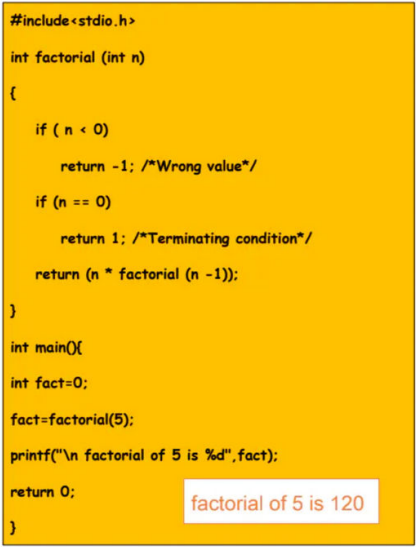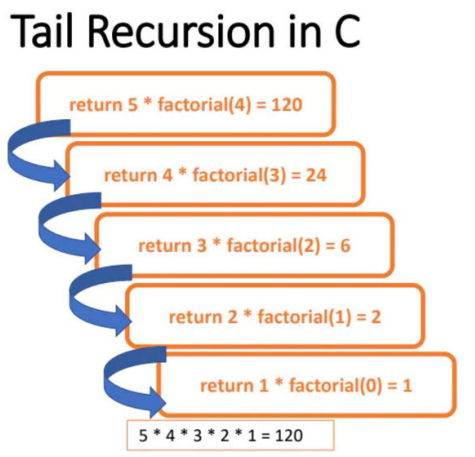### Recursion in C

• When a function calls itself it is known as recursion.

recursionfunction(){

recursionfunction(); //calling self function

}

• The function which calls the same function is known as a recursive function.

• A function that calls itself and doesn’t perform any task after the function call is known as tail recursion.

• In tail recursion,we generally call the same function with a return statement.

Example of recursion:

Finding out factorial using recursion: